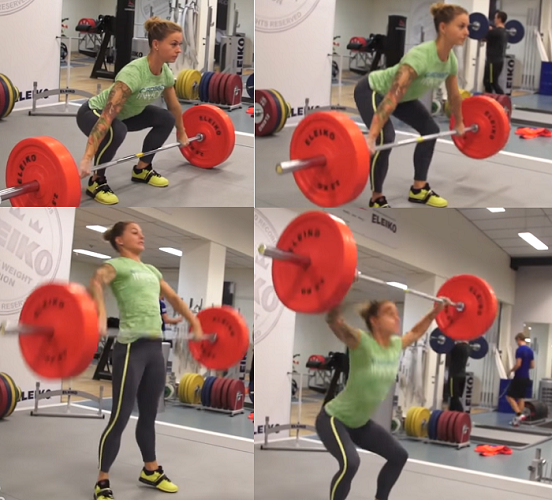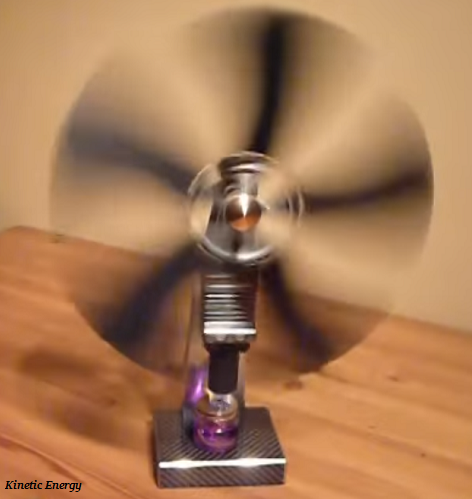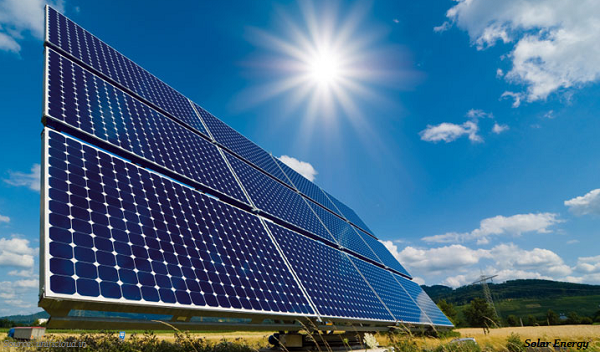# Physics - Work and Energy

## Introduction

• When acting (applying force), there is a displacement of the point of application in the direction of the force, is known as work.

• The term work was first introduced by the French mathematician GaspardGustave Coriolis in 1826.• Work done by a force acting on the object is equal to the magnitude of the force multiplied by the distance moved in the direction of the force and it is calculated as −

• Work done (W) = Force (F) × Displacement (s)

• Work has the only magnitude and no direction.

• The SI unit of work is the joule (J).

## Energy

• Energy can be converted in form, but cannot be created or destroyed. For example, producing electric energy from solar energy, etc.

• The object which does the work loses energy and the object on which the work is done gains energy.

• Further, the object that possesses energy can exert a force on another object to transfer energy from former to later.

• The energy possessed by an object is therefore measured in terms of its capacity of doing work.

• The SI unit of energy is joule (J).

## Forms of Energy

• Following are the major forms of the energy −

• Potential energy

• Kinetic energy

• Heat energy

• Chemical energy

• Electrical energy

• Light energy

• Let’s discuss each one in brief

## Potential Energy

• The energy, possessed by a body by virtue of its position relative to others, is known as Potential energy.

• So, potential energy is the stored energy in an object. For example, gravitational potential energy, elastic potential energy, electrical potential energy, etc.

• The SI unit of potential energy is joule (J).

• The term potential energy was introduced by Scottish engineer and physicist William Rankine.

## Kinetic Energy

• The energy that an object possesses because of its motion, is known as kinetic energy.• The running/moving body maintains its kinetic energy unless its speed changes (increases or decreases).

• The SI unit of kinetic energy is joule (J).

## Heat Energy

• Heat is a form of energy transferred spontaneously from a hotter to a colder body.

## Chemical Energy

• The potential of a chemical substance to experience a transformation through a chemical reaction and transform other chemical substances is known as chemical energy. E.g. Breaking or making of chemical bonds, batteries, etc.

• The chemical energy of a (chemical) substance can be converted to other forms of energy by a chemical reaction. E.g., green plants convert solar energy to chemical energy (commonly of oxygen) by the process of photosynthesis.

## Electrical Energy

• The energy, derived from electric potential energy or kinetic energy, is known as electrical energy.

• Electricity is normally produced by electromechanical generators at a power station.

• The electromechanical generators primarily are driven by heat engines fueled by the kinetic energy of flowing water and wind.

• The electromechanical generators are also driven by heat engines fueled by chemical combustion or nuclear fission.

## Light Energy

• Light is a form of electromagnetic radiation.

• Light energy most likely is the only form of energy that we can really see.• Light is transferring energy through the space in a natural way. E.g. solar energy.

## Law of Conservation of Energy

• Law of conservation of energy states that energy can neither be created nor be destroyed; however, it can be only transformed from one form to another.

• According to the law of conservation of energy, the total energy before and after the transformation remains the same.

• The law of conservation of energy remains valid in all conditions and locations and for all kinds of transformations.